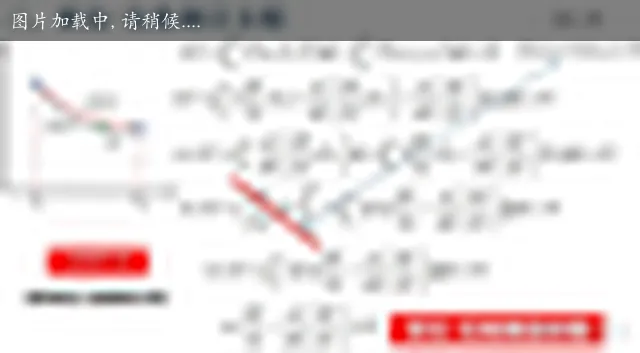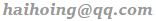### P1 傅里叶变换、傅立叶级数和频谱 15:46

https://blog.csdn.net/weixin_48524215/article/details/122802096

2023.09.02

### P2 拉普拉斯变换直观理解 19:56

Fourier 变换时常会遇到的问题就是积分不收敛，当加上那个指数下降的项之后大多情形都解决了。

$\begin{array}{l} F(s)=\int_{0}^{\infty} f(t) \mathrm{e}^{-s t} \mathrm{~d} t \quad \operatorname{Re}[s]>\sigma_{0} \\ F(\mathrm{j} \omega)=\int_{-\infty}^{\infty} f(t) \mathrm{e}^{-\mathrm{j} \omega t} \mathrm{~d} t \end{array} \\$

### P5 欧拉-拉格朗日方程 Euler-Lagrange equation explained intuitively - Lagrangian Mechanics 18:23### P29 拉普拉斯方程 05:31

If you have any questions or feedback, please reach out.